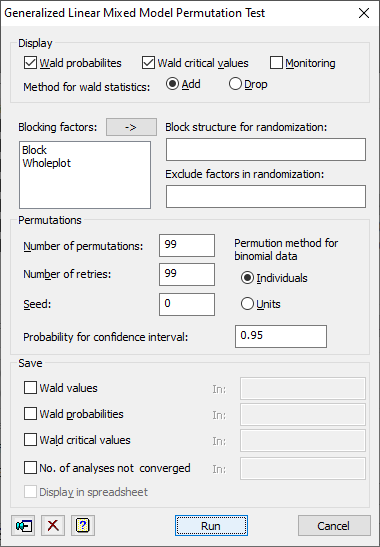1. Home
2. Generalized Linear Mixed Model Permutation Test

# Generalized Linear Mixed Model Permutation Test

This dialog allows you to assess the significance of the fixed terms in a generalized linear mixed model using a permutation test done by the GLPERMTEST procedure.## Display

 Wald probabilities probabilities for the fixed terms, estimated from the locations of their Wald statistics within the sets obtained from the permuted data sets Wald critical values critical values for the Wald statistics, estimated by quantiles within the sets from the permuted data sets Monitoring Monitoring information, showing the progress of the analysis

## Method for Wald statistics

This option selects the type of test used.

 Add the tests are for adding terms sequentially to the model Drop the tests are for dropping terms from the full fixed model

If Drop is selected, tests will only be made for terms which are not marginal to other terms in the model, e.g. for A + B + A.B, only the A.B termwill be tested are A and B are marginal to A.B, and so cannot be dropped from the full model.

## Blocking factors

This is a list of the factors used in the random model. These can be used in the Block structure for randomization or Exclude factors in randomization fields. Double clicking an item in the list will enter it into the last field that had focus. Clicking thebutton will put the selected items in the last field that had focus.

## Block structure for randomization

If the data are from a designed experiment, you may need to use the field to specify a block model to define how to do the randomization. See the RANDOMIZE directive for further details.

## Exclude factors in randomization

This option can be used to restrict the randomization so that one or more of the factors in the block model are not randomized. The blocking factors not to be randomized can be entered in a comma or space separated list.

## Number of permutations

This option specifies the number of permutation samples that are performed, default 99 (as well as the “null” permutation where the data keep their original order).

## Number of retries

This option specifies the number of extra permutations that are performed when the REML model does not converge for a permutation, default 99.

## Seed

Specifies the seed for the random number generator used to make the permutations; default 0 continues from the previous generation or (if none) initializes the seed automatically.

## Permutation method for binomial data

This option controls how the permutations are done for binomial data. The original data set will have contained a set of units, each recording a number of “successes” obtained from an observed number of individuals. The options are:

 Individuals expand the data set to contain the individuals, and permute these – this is the recommended method Units permute the units as a whole

## Probability for confidence interval

This gives the probability that the confidence interval covers the true value. This should be a value between 0 and 1 and if it is too close to 1 then the interval will not be well estimated unless the number of bootstraps is large.

## Save

This lets you save results from the from the GLPERMTEST analysis in Genstat data structures. After selecting the appropriate boxes, you need to type the names for the identifiers of the data structures into the corresponding In: fields.

 Wald values Pointer to variate Wald values for each permutation for each term in the fixed model Wald probabilities Pointer to scalars Wald probabilities for each term in the fixed model Wald critical values Pointer to scalar Wald critical values for each term in the fixed model No. of analyses not converged Scalar The number of analyses for which the model fitting did not converge

If there is a single random term being tested, the saved structures for the Wald results will be scalars, otherwise they will be pointers.